# NCERT Solutions for Class 9 Science Chapter 11 - Work and Energy

##### Question 1:

A force of 7 N acts on an object. The displacement is, say 8 m in the direction of the force. Let us take it that the force acts on the object through the displacement. What is the work done in this case?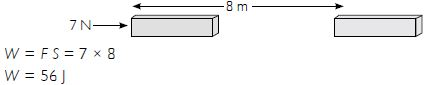##### Question 2:

When do we say that work is done?

Work is said to be done when the force applied move the body through some distance.

##### Question 3:

Write the expression for the work done when a force is acting on an object in the direction of its displacement.

If F is the force acting in the direction of displacement S, then the work done is W = F S.

##### Question 4:

Define 1 J of work

Work done on a body is said to be 1 J if a force of 1 N applied on a body displaces it by 1 m in the direction of force.

##### Question 5:

A pair of bullocks exerts a force of 140 N on a plough. The field being ploughed is 15 m long. How much work is done to plough the length of the field?

W = F S = 140 x 15
W = 2,100 J

##### Question 6:

What is kinetic energy of an object?

Kinetic energy of an object is the energy possessed by it due to its motion.

##### Question 7:

Write an expression for kinetic energy of an object.

Kinetic energy of an object of mass m moving with velocity u is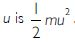.. If its velocity is increased from u to v, then kinetic energy of the object =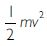\ The increase in kinetic energy =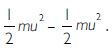##### Question 8:

The kinetic energy of an object of mass m is moving with a velocity of 5 ms-1 is 25 J. What will be its kinetic energy when its velocity is doubled? What will be its kinetic energy when its velocity is increased three times?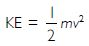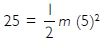or m = 2 kg
When velocity is doubled i.e.,
v = 2 × 5 ms-1 = 10 ms-1, then,

\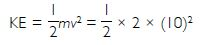= 100 J
When velocity is increased to three times,
v = 3 × 5 = 15 ms-1

\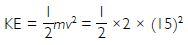= 225 J

##### Question 9:

What is power?

Power is the rate of doing work.
It is a scalar quantity. The unit of power is watt.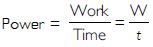##### Question 10:

Define 1 watt of power.

Power of an agent is 1watt if work is done at the rate of 1 joule per second.
Watt = joule/second
1 W = 1 Js-1 = 107 ergs-1.

##### Question 11:

A lamp consumes 1,000 J of electric energy in 10 s. What is its power?

W = 1,000 J; t = 10 s; P =?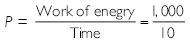P = 100 watt

##### Question 12:

Look at the activities listed below. Reason out whether or not work is done in the light of your understanding of the term ‘work’.
(i) Suma is swimming in a pond.
(ii) A donkey is carrying a load on its back.
(iii) A wind mill is lifting water from a well.
(iv) A green plant is carrying out photosynthesis.
(v) An engine is pulling a train.
(vi) Food grains are getting dried in the sun.
(vii) A sail boat is moving due to wind energy.

(i) Suma is doing negative work while swimming. She is applying force in the backward direction and is moving in the forward direction. Since her displacement is in a direction opposite to the force applied, she is doing negative work.
(ii) The donkey moving horizontally is not doing any work. Force is being applied in the vertical direction but displacement is in the horizontal direction only. Hence, no work is done because displacement and force are in perpendicular direction.
(iii) Positive work is being done because in lifting water, as displacement and force are in a vertically upward direction.
(iv) A green plant carrying photosynthesis does no work since neither there is a force applied nor does any displacement occur.
(v) An engine pulling a train is doing positive work since the displacement is in the direction of force applied.
(vi) No work is done on food grain because neither there is a force applied nor does any displacement occur.
(vii) Positive work is being done since the force and displacement are in the same direction.

##### Question 13:

An object thrown at a certain angle to the ground moves in a curved path and falls back to the ground. The initial and the final points of the path of object lie on the same horizontal line. What is the work done by the force of gravity on the object?

Zero, since the net vertical distance covered in the direction of force of gravity is zero.

##### Question 14:

A battery lights a bulb. Describe the energy changes involved in the process.

In a battery, the chemical energy is first converted to electrical energy. Electrical energy supplied by the battery is being converted into heat and light energy.

##### Question 15:

A certain force acting on a 20 kg mass change its velocity from 5 ms-1 to 2 ms-1. Calculate the work done by the force.

Here m = 20 kg; u = 5 ms-1; v = 2 ms-1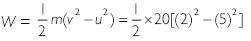W = 10 [4 - 25] = 10 (- 21)
W = - 210 J
Work done is negative because an opposing force is being applied.

##### Question 16:

A mass of 10 kg is at a point A on a table. It is moved to a point B. If a line joining A and B is horizontal, what is the work done on the object by gravitational force? Explain your answer.

A force of gravity is being applied in the downward vertical direction and the displacement is in the horizontal direction. There is no displacement in the direction of force applied; therefore, work done is zero.

##### Question 17:

The potential energy of a freely falling object decreases progressively. Does this violate the law of conservation of energy? Why?

It does not violate the law of conservation of energy. Potential energy goes on decreasing as the body falls down; so is its velocity and hence, the kinetic energy increases.
Kinetic energy increases by the same amount as potential energy has decreased keeping the total mechanical energy conserved.

##### Question 18:

What are the various energy transformations that occur when you are riding a bicycle?

The food which the cyclist has eaten has chemical energy. While cycling, the cyclist transfers this chemical energy into mechanical energy. In turn, mechanical energy of the cyclist does work against rolling friction between the cycle and the ground. In doing work against friction, he converts part of the energy into sound energy and heat energy.

##### Question 19:

Does the transfer of energy takes place when you push a huge rock with all your might and fail to move it? Where is the energy you spent going?

Since we do not find evidence of any displacement, work appears to be zero. The work done by you on the object is zero; however, work done by you on your own body is not zero.
While pushing the rock, you had to stretch your muscles, heart had to pump more blood and in making these changes, your energy is definitely lost.

##### Question 20:

A certain household has consumed 250 units of energy during a month. How much energy is this in joules?

Practical unit of electric energy is kWh.

1 kWh = 1,000 W × 1 h =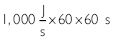1 kWh = 3.6 × 106 J
250 kWh = 250 × 3.6 × 106 J = 9 × 108 J
\ Energy spent in a month = 9 × 108 J.

##### Question 21:

An object of mass 40 kg is raised to a height of 5 m above the ground. What is its potential energy? If the object is allowed to fall, find its kinetic energy when it is half-way down.

Here m = 40 kg; h = 5 m; g = 10 ms-2
PE = mgh = 40 × 10 × 5
\ PE = 2,000 J
When it is half way down i.e., at a height 5/2 = 2.5 m
PE = mgh = 40 × 10 × 2.5 = 1,000 J
KE = Total energy - P.E. (at half way)
= 2,000 - 1,000 = 1,000 J

##### Question 22:

What is the work done by the force of gravity on a satellite moving round the earth? Justify your answer.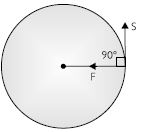Work done is zero because the displacement is in a direction perpendicular to the direction of centripetal force. The centripetal force is acting towards the centre whereas the displacement is always acting tangential to the circular path (i.e., at 90$°$). There is no displacement in the direction of force and hence, work done is zero.

##### Question 23:

Can there be displacement of any object in the absence of any external force? Think and discuss this question with your friends and teacher.

Yes, if the body is initially in uniform motion, it will continue to move uniformly in a straight line (i.e., it will continuously be displaced) in the absence of an external force in accordance with Newton’s first law of motion.

##### Question 24:

A person holds a bundle of hay over his head for 30 minutes and gets tired. Has he done some work or not? Justify your answer.

Since there is no displacement of hay in the direction of force applied, therefore, work done by him on hay is zero.

##### Question 25:

An electric heater is rated 1,500 W. How much energy does it use in 10 hours?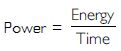or Energy = Power × Time
E = Pt = 1,500 W × 10 h = 15,000 Wh
or E = 15 kWh
Hence, the energy consumed by the heater in 10 h is 15 kWh.

##### Question 26:

Illustrate the law of conservation of energy by discussing the energy changes which occur when we draw a pendulum bob to one side and allow it to oscillate. Why does the bob eventually come to rest? What happens to its energy eventually? Is it a violation of the law of conservation of energy?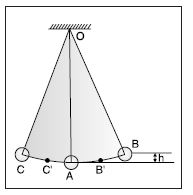At mean position i.e., at A, potential energy, PE, is zero. When it is displaced from A to B, it gains PE. If B is at a distance h vertically above A, the bob has a PE of mgh. When it is released and it returns from B to say B’, it loses some of the PE and has a corresponding gain of kinetic energy (KE). On reaching A, its whole of PE (at B) gets converted into KE. (at A). The speed of the bob (u) at A is: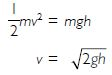Due to this speed, the bob overshoots A and reaches C’ where its energy is partly kinetic and partly potential but the sum of KE and PE is the sum of KE at A and PE at B. When it reaches C, it whole energy is PE.
The bob comes to rest after some time because of the resistance offered by air to its motion and work done against friction.
There is no violation of law of conservation of energy. The energy initially possessed by the bob is mostly converted to the heat energy due to the resistance of air and friction at point of support. A small fraction of energy is also converted into sound energy. However, the total energy of the pendulum and the surrounding system remain conserved.

##### Question 27:

An object of mass m is moving with a constant velocity v. How much work should be done on the object in order to bring the object to rest?

Let the object of mass m be initially moving with velocity v and finally be brought to rest by the application of an opposing force F. Let it stops after covering a distance S.
v = 0; a =?, u = v
Using v2 - u2 = 2aS
(o)2 - (v)2 = 2aS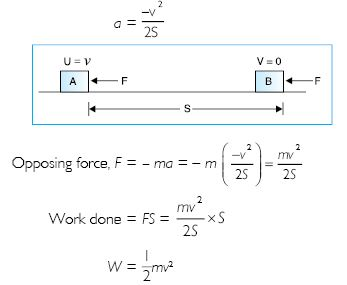##### Question 28:

Calculate the work required to be done to stop a car of 1,500 kg moving at a velocity of 60 kmh-1.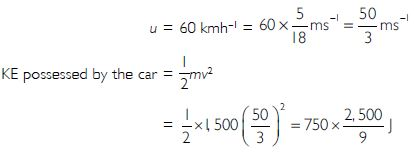= 208,333 J = 2.08 × 105 J
To stop the car, the work done on the car is in the opposite direction and should be equal to KE possessed by it i.e.,
W = 2.08 × 105 J

##### Question 29:

In each of the following, a force F is acting on an object of mass m. The direction of displacement is from west to east shown by longer arrow. Observe the diagrams carefully and state whether the work done by the force is negative, positive or zero.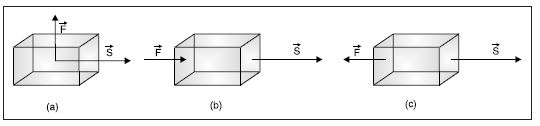(a) In [Fig. (a)], Displacement is in a direction perpendicular to direction of force applied, therefore, work done is zero. There is no displacement in the direction of force.
(b) Work done is positive since the force and displacement are in the same direction [Fig. (b)].
(c) Work done is negative since the displacement is in a direction opposite to the direction of force [Fig. c)].

##### Question 30:

Soni says that the acceleration in an object could be zero even when several forces are acting on it. Do you agree with her? Why?

Yes, acceleration can be zero even when a large number of forces are acting on a body, but their resultant is zero. As F = ma; If F = 0, then ma = 0, m cannot be zero, therefore, a = 0.
Illustrations:
(i) If two equal and opposite forces are acting on an object, the acceleration of the object is zero.
(ii) If three forces are simultaneously acting on an object and can be represented in magnitude and direction by the three sides of the triangle in the same order, the body is in equilibrium and will have zero acceleration.
(iii) Even with more than three forces acting on a body, it could have zero acceleration if the resultant of all forces is zero.

##### Question 31:

Find the energy in kWh consumed in 10 hours by four devices of power 500 W each.